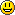### Programming Reference/Librarys

Q&A is closed

arduino:bitwise_operators:bitwisecompoundor# compound bitwise OR (|=)

### Description

The compound bitwise OR operator (|is often used with a variable and a constant to “set” (set to 1) particular bits in a variable.

### Syntax:

`x |= y;   // equivalent to x = x | y; `

### Parameters

x: a char, int or long variable
y: an integer constant or char, int, or long

### Example:

First, a review of the Bitwise OR (|) operator

```   0  0  1  1    operand1
0  1  0  1    operand2
----------
0  1  1  1    (operand1 | operand2) - returned result```

Bits that are “bitwise ORed” with 0 are unchanged, so if myByte is a byte variable,

myByte | B00000000 = myByte;

Bits that are “bitwise ORed” with 1 are set to 1 so:
myByte | B11111111 = B11111111;

Consequently - to set bits 0 & 1 of a variable, while leaving the rest of the variable unchanged, use the compound bitwise OR operator (|with the constant B00000011

```   1  0  1  0  1  0  1  0    variable
0  0  0  0  0  0  1  1    mask
----------------------
1  0  1  0  1  0  1  1

variable unchanged
bits set
```

Here is the same representation with the variables bits replaced with the symbol x

```   x  x  x  x  x  x  x  x    variable
0  0  0  0  0  0  1  1    mask
----------------------
x  x  x  x  x  x  1  1

variable unchanged
bits set```

So if:

```myByte =  B10101010;

myByte |= B00000011 == B10101011;```

See Also * &= (compound bitwise and) * & (bitwise and) * | (bitwise or)

Source: arduino.cc

## on the occasion of the current invasion of Russia in Ukraine